# Number and Algebra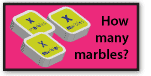Introduction to algebra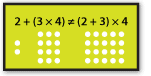The laws of arithmetic and their use in algebra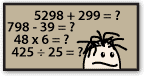Mental computation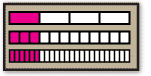Equivalent fractions and the use of the number line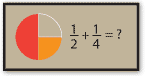Addition and subtraction of fractions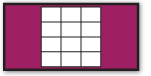Multiplication and division of fractionsMultiplication and division of decimalsSquare roots and square numbersRounding decimalsExpressing one quantity as a fraction of a second

# Measurement and Geometry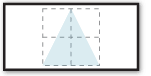The Cartesian plane and plotting points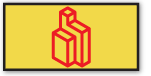Geometric drawing including representation of simple solids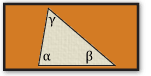Geometric reasoning including parallel lines and angle sum of a triangleTransformations of the plane

# Statistics and Probability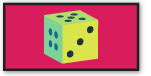Probability